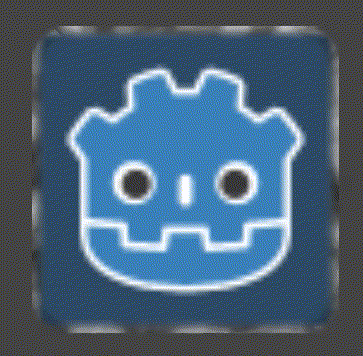+1 vote

Hello everybody,

I'm trying to create an sketchy or animated 2D outline shader like this one:

I tried the mentioned shader on some 2D-Sprites and meshes but without success.
Can anyone point me in the right direction how to make it work in 2D or how to create a similar one?

I'm quite new to shader programming. I already managed to draw an simple outline but I have no idea what is the best way to animate it? Perhaps with applying some time depending noise?

Any help is really appreciated.

Best regards

Edit: This is the last version of a shader that I have adapted but it is not working very well ;) :

``````shader_type canvas_item;

uniform sampler2D NOISE_PATTERN;
uniform vec2 vieportSize = vec2(44,44);

float random (in vec2 st) {
return fract(sin(dot(st.xy, vec2(12.9898,78.233))) * 43758.5453123);
}

float noise (in vec2 st) {
vec2 i = floor(st);
vec2 f = fract(st);

float a = random(i);
float b = random(i + vec2(1.0, 0.0));
float c = random(i + vec2(0.0, 1.0));
float d = random(i + vec2(1.0, 1.0));

vec2 u = f*f*(3.0-2.0*f);

return mix(a, b, u.x) +
(c - a)* u.y * (1.0 - u.x) +
(d - b) * u.x * u.y;
}

void fragment() {
vec2 st = FRAGCOORD.xy/vieportSize.xy;

vec2 pos = vec2(st*5.0);

float n = noise(pos);

vec4 tmpColor = texture(TEXTURE, UV);
tmpColor += smoothstep(random(UV*TIME),.2,noise(st*10.));
tmpColor -= smoothstep(.35,.4,noise(st*10.));

COLOR = tmpColor;
COLOR.a = texture(TEXTURE, UV).a;
}
``````
Godot version 3.2.3
in Engine
edited

It is always handy to show what you did so far. I would first create the 3D variant in a 3D scene then start a 2D scene. You need a canvas_item shader to start with :-p

I talked to QbieShay on Discord (the creator of the original shader) and she said that it might not translate well to 2D since "it is substantially based on Fresnel and front face culling" "but perhaps with an outline mesh + a normal map + double pass".

Did you manage to reproduce my 2D version? And please mark the answer as the answer when applicable :)

+1 vote

Configure a OpenSimplexNoise somewhere on that page I came to mixing the noise on the UV borders. Not sure that is what you are after :-/

Animated GIFand better quality MOV 2D result

``````shader_type canvas_item;

uniform sampler2D outline_noise_tex;

void fragment() {
vec2 n_uv = UV - 0.5;
vec2 rotated_uv = vec2(cos(TIME) * n_uv.x + sin(TIME) * n_uv.y, sin(TIME) * n_uv.x - cos(TIME) * n_uv.y);
vec4 noise = texture(outline_noise_tex, rotated_uv);
vec4 original = texture(TEXTURE, UV);

// borders in UV space are maxed out on 0.5
vec2 normalised = abs(n_uv);
// this creates a circle
//float delta = length(normalised);

// This adds border around squared
float delta = max(normalised.x, normalised.y);

COLOR.xyz = mix(original.rgb, noise.rgb, smoothstep(0.45, 0.5, delta));
COLOR.a = original.a;
//COLOR.a = mix(original.a, 0.0, smoothstep(0.9, 1.0, delta));
}
``````
by (638 points)
selected by

I added rotation to the noise texture which makes it better :-)

Thank you, this will do it :)

Nice you can use this :-)